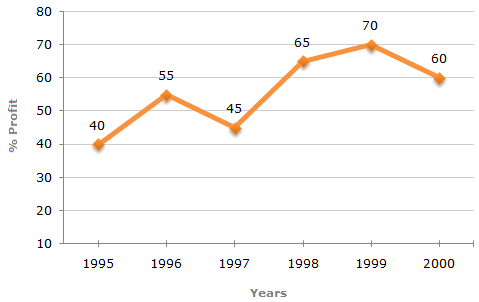### Line Chart - 5

The following line graph gives the annual percent profit earned by a Company during the period 1995 - 2000.

Percent Profit Earned by a Company Over the Years.

 %Profit = Income - Expenditure x 100 Expenditure1.  If the income in 1998 was ₹ 264 crores, what was the expenditure in 1998?

 A. ₹ 104 crores B. ₹ 145 crores C. ₹ 160 crores D. ₹ 185 crores E. None of these
2.  If the expenditures in 1996 and 1999 are equal, then the approximate ratio of the income in 1996 and 1999 respectively is?

 A. 1 : 1 B. 2 : 3 C. 13 : 14 D. 9 : 10 E. Cannot be determined
3.  If the profit in 1999 was ₹ 4 crores, what was the profit in 2000?

 A. ₹ 4.2 crores B. ₹ 6.6 crores C. ₹ 6.8 crores D. Cannot be determined E. None of these
4.  In which year is the expenditure minimum?

 A. 2000 B. 1997 C. 1996 D. Cannot be determined E. None of these
5.  What is the average profit earned for the given years?

 A. 50(2/3) B. 55(5/6) C. 60(1/6) D. 335 E. None of these